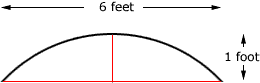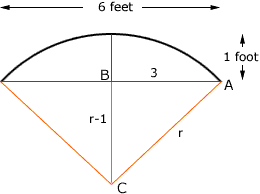Quandaries and Queries My name is Daryl and here is my question.  I am tying to find the arc length of a line 6' and the vertex of the arc 1' off of the line. that is all that is known The attached PDF file has a drawing of what I'm talking about.   I did a search in your archive and found a question almost exact to mine, however there was inadequate information and it was not answered. Hi Daryl, The extra piece of information you have is enough to find the length of the arc.Join the endpoints of the arc to the center of the circle and let r be the radius of the circle. The diagram is thenThe triangle ABC is a right triangle and hence, by Pythagoras' Theorem 32 + (r - 1)2 = r2 or 9 + r2 - 2 r + 1 = r2 Thus r = 5. The angle BCA is thus cos-1(4/5) = 0.6435 radians and hence the angle at the center of the circle subtended by the arc is 1.287 radians. The length of the arc is given by arc length = rtheta where theta is the angle subtended by the arc in radians. Thus arc length = 51.287 = 6.436 feet. Penny Go to Math Central